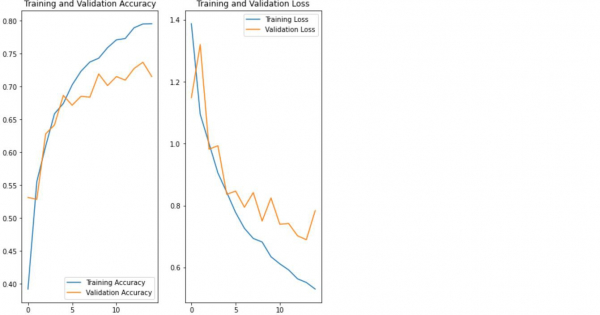# How can Tensorflow be used to visualize the results of the model?

The flower dataset, after applying augmenting and dropout methods (to avoid overfitting) can be visualized using ‘matplotlib’ library. It is done using the ‘plot’ method.

We will use the Keras Sequential API, which is helpful in building a sequential model that is used to work with a plain stack of layers, where every layer has exactly one input tensor and one output tensor.

A neural network that contains at least one layer is known as a convolutional layer. We can use the Convolutional Neural Network to build learning model.

An image classifier is created using a keras.Sequential model, and data is loaded using preprocessing.image_dataset_from_directory. Data is efficiently loaded off disk. Overfitting is identified and techniques are applied to mitigate it. These techniques include data augmentation, and dropout. There are images of 3700 flowers. This dataset contaisn 5 sub directories, and there is one sub directory per class. They are:

daisy, dandelion, roses, sunflowers, and tulips.

We are using the Google Colaboratory to run the below code. Google Colab or Colaboratory helps run Python code over the browser and requires zero configuration and free access to GPUs (Graphical Processing Units). Colaboratory has been built on top of Jupyter Notebook.

When the number of training examples is small, the model learns from noises or unwanted details from training examples. This negatively impacts the performance of the model on new examples.

When dropout is applied to a layer, it randomly drops out a number of output units from the layer when the training is going on. This is done by setting the activation function to 0. Dropout technique takes a fractional number as the input value (like 0.1, 0.2, 0.4, and so on). This number 0.1 or 0.2 basically indicates that 10 percent or 20 percent of the output units are randomly from the applied layer.

Data augmentation generates additional training data from the existing examples by augmenting them with the help of random transformations that would yield believable-looking images. Following is an example:

## Example

print("Visualizing the data after performing data augmentation and dropout")
print("Accuracy is being calculated")
acc = history.history['accuracy']
print("Loss is being calculated")
val_acc = history.history['val_accuracy']
loss = history.history['loss']
val_loss = history.history['val_loss']
epochs_range = range(epochs)
print("The results are being visualized")
plt.figure(figsize=(8, 8))
plt.subplot(1, 2, 1)
plt.plot(epochs_range, acc, label='Training Accuracy')
plt.plot(epochs_range, val_acc, label='Validation Accuracy')
plt.legend(loc='lower right')
plt.title('Training and Validation Accuracy')
plt.subplot(1, 2, 2)
plt.plot(epochs_range, loss, label='Training Loss')
plt.plot(epochs_range, val_loss, label='Validation Loss')
plt.legend(loc='upper right')
plt.title('Training and Validation Loss')
plt.show()

## Output

Visualizing the data after performing data augmentation and dropout
Accuracy is being calculated
Loss is being calculated
The results are being visualized## Explanation

• The data is visualized using ‘matplotlib’ library.
• The accuracy and loss associated with training the model is calculated.
• The training accuracy and validation accuracy are displayed on the console.

Updated on: 22-Feb-2021

272 Views